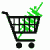February 7, 2023

# How to convert VA to Watts and KVA to Kilowatts

## Convert 3-phase Amps to KVA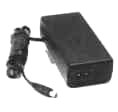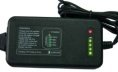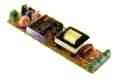3-phase inverters 115VAC to 415VAC output DC input ATX computer power supplies Power Supplies and Adapters Battery chargers for cells or packs 12V and 24V input electronic ballast for 20W and 40W fluorescent lamps
Custom design and manufacture of state-of-the-art power supplies, battery packs, battery chargers, AC Adapters, and power supplies for OEMs in a Hurry

## Basics of AC power calculation

Since watts is volts times amps, what is VA? VA (or volt-amps) is also volts times amps, the concept however has been expanded and extended cover some of the subleties of AC power.

For DC current:

## VA = Watts (DC current).

In AC if the volts and amps are in phase (for example a resistive load) then the equation is also

where V is the RMS voltage and A the RMS amperage.

But here is the difference. In AC, the volts and amps are not always in phase (meaning that the peak of the voltage curve is does not happen at the peak of the current curve). Think of pushing a swing. The greatest force is applied when the swing stops at the peak, but the greatest velocity is at the bottom of the arc. When you have reactive circuit elements the current can't keep up with the voltage, but lags behind. So in AC, if the volts and amps are not precisely in phase you have to calculate the watts by multiplying the volts times the amps at each moment in time and take the average over time, i.e. the integral of V*A dt over a full cycle. The ratio between the VA (i.e. rms volts time rms amps) and Watts is called the power factor PF.

In other words, volt-amps x power factor = watts. Similarly, KVA*PF = KW,
Or kilovolt-amps times power factor equals kilowatts.

When you want to know how much the electricity is costing you, you use watts. When you are specifying equipment loads, fuses, and wiring sizes you use the VA, or the rms voltage and rms amperage. This is because VA considers the peak of both current and voltage, without taking into account if they happen at the same time or not.

## Finding the Power Factor

How do you find the power factor? This isn’t easy. The idea is that you multiply the amps times the volts for every increment of time and take the sum and average. You then divide this by the power you would have calculated if the amps and volts are exactly in phase. Since the voltage and current can be out of phase (which is the whole point of power factor) the power factor can be anywhere between zero and one. For computer power supplies and other supplies that are power factor corrected the power factor is usually over 90%. For high power motors under heavy load the power factor can be as low as 35%.

Industry standard rule-of-thumb is that you plan for a power factor of 60%, which somebody came up with as a kind of average conservative power factor.

## Converting VA to Amps

How to convert VA to amps? Use the following formula: A = (Va * Pf)/V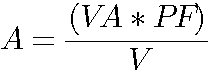Where A stands for the RMS amps, VA stands for volt-amps, V stands for RMS volts and PF stands for the power factor.

## Converting VA to Volts

How to convert VA to volts? Use the following formula: V=(Va*Pf)/A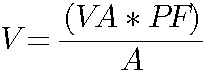Where V stands for RMS volts, A stands for the RMS amps, VA stands for volt-amps, and PF stands for the power factor.

## What is KVA?

KVA is just kilovolt-amps, or volts times amps divided by 1000:

Where KVA stands for kilovolt-amps, KW stands for kilowatts, and PF stands for the power factor.

Keep the factor of 1000 straight when dealing with mixed units:

VA·PF = 1000·KW (Kilowatts to VA)

The Following equations can be used to convert between amps, volts, and VA. To convert between kilovolt-amps, kilowatts, and kiloamps, keep track of the factor of 1000.

Convert VA to Amps (at a fixed voltage)
Convert KVA to KW (kilovolt-amps to kilowatts)
Convert KVA to Amps (at a fixed voltage)
Converting Watts to KVA (watts to kilovolt-amps)
Convert Amps to VA (at a fixed voltage)
Convert VA to Volts (at a fixed current)
Convert Volts to VA (at a fixed current)
Convert Volts to Amps (at a fixed VA)
Convert Amps to Volts (at a fixed VA)

## Converting VA to Amps (voltage fixed)

The conversion of VA to Amps is governed by the equation Amps = VA·PF/Volts)

For example 12 VA·0.6/(12 volts) = 0.6 amp

## Converting KVA to KW (Kilovolt-amps to Kilowatts)

The conversion of KVA to KW is governed by the equation KVA = KW/PF)

For example, if the power factor is 0.6
120 KVA·0.6 = 72 Kilowatts

## Converting Watts to KVA (watts to kilovolt-amps)

The conversion of W to KVA is governed by the equation KVA=W/(1000*PF)

For example 1500W/(1000*0.83) = 1.8 kVA (assuming a power factor of 0.83)

Converting Amps to VA (voltage fixed)

The conversion of Amps to VA is governed by the equation VA = Amps · Volts/PF

For example 1 amp * 110 volts/0.6 = 183 VA

## Converting Amps to KVA (voltage fixed)

The conversion of Amps to KVA is governed by the equation KVA = Amps · Volts/(1000·PF)

For example 100 amp * 110 volts/(1000*0.6) = 18.3 KVA

## Converting VA to Volts (current fixed)

The conversion of VA to Volts is governed by the equation Volts = VA·PF/Amps

For example 100 VA · 0.6/10 amps = 6 volts

## Converting Volts to VA (current fixed)

The conversion of Volts to VA is governed by the equation VA = Amps · Volts/PF

For example 1.5 amps * 12 volts/0.6 = 30 VA

## Converting Volts to Amps at fixed VA

The conversion of Volts to Amps is governed by the equation Amps = VA·PF/Volts

For example 120 VA* 0.6 /110 volts = 0.65 amps

## Converting Amps to Volts at fixed VA

The conversion of Amps to Volts is governed by the equation Volts = VA·PF/Amps

For Example, 48 VA · 0.6 / 12 Amps = 2.4 Volts

## Converting Amps to Watts and VA in 3-Phase Power

In three phase power, the basic equation is multiplied by the square root of 3, or 1.732
So VA = V * I * 1.732
W = V * I * 1.732 * PF

Thus a 3-phase motor running at 6 amps and 460 volts would require 5000VAThank you for considering PowerStream

Volt x amps, how to calculate volt-amps, kilovolt-amps, amps to KVA conversion, electrical KVA, KVA to KW, KVA calculations, what is kva? Convert amps to VA. Convert VA to amps. Convert VA to volts, convert volts to VA, convert amps to volts at fixed wattage. How to convert VA to amps. How do I convert amps to VA? Amps converting VA. Volt to VA conversion.

 PowerStream Technology 1163 South 1680 West Orem, Utah 84058 USA Phone: 801-764-9060 Fax: 801-764-9061 © Copyright 2000, 2002, 2003, 2004, 2005, 2006, 2008,2009, 2010, 2011, 2012, 2013, 2014, 2015, 2016, 2017, 2018, 2019, 2020, 2021, 2022 Lund Instrument Engineering, Inc. All rights reserved. Google Author Verification